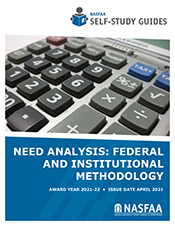# Need Analysis: 2022-23

The Need Analysis Self-Study Guide reviews the calculation of a student's expected family contribution (EFC), and includes a discussion of the regular and simplified formulas as well as recalculations. Bonus material: Included with this Guide is an optional lesson on institutional methodology, developed in collaboration with the College Board. Completion of this self-study guide qualifies individuals to take the corresponding NASFAA Professional Credential test.

Available as a PDF only.

Free (Value Members and Value Plus Members)
\$109.00 (Members)
\$218.00 (Non-Members)

### Learning ObjectivesLesson 1: Introduction to Need Analysis

• Understand what is need analysis;
• Know a brief history and the evolution of need analysis; and
• Understand the underlying principles of need analysis.

Lesson 2: The Regular Formula

• Recognize the three models used to calculate the EFC;
• Identify the model which should be used to calculate a student’s EFC; and
• Learn the steps used to calculate the EFC using the regular formula.

Lesson 3: Simplified Formulas

• Recognize the differences between the regular formula and the simplified formulas;
• Be able to identify which models qualify for the simplified formulas;
• Understand the eligibility criteria for an Automatic Zero EFC; and
• Understand the eligibility criteria for the Simplified Needs Test.

Lesson 4: Recalculating the EFC

• Understand how to recalculate the EFC for enrollment periods other than nine months; and
• Learn approaches for calculating the EFC for summer periods of enrollment.